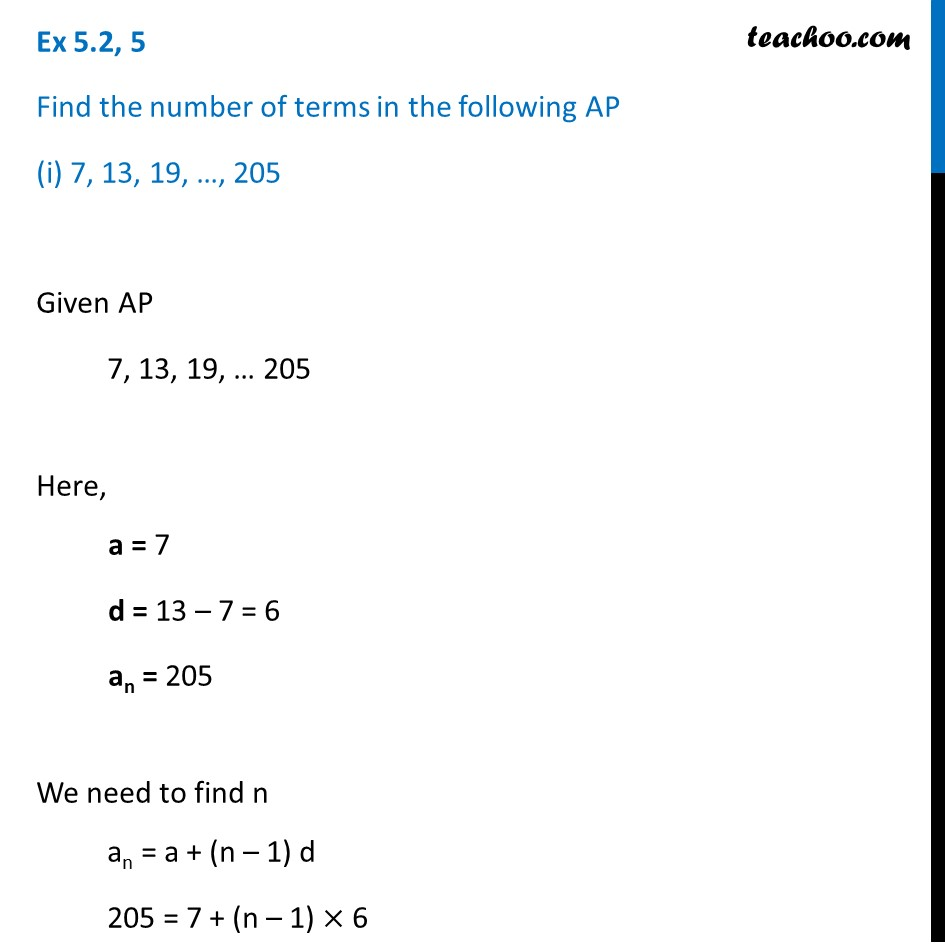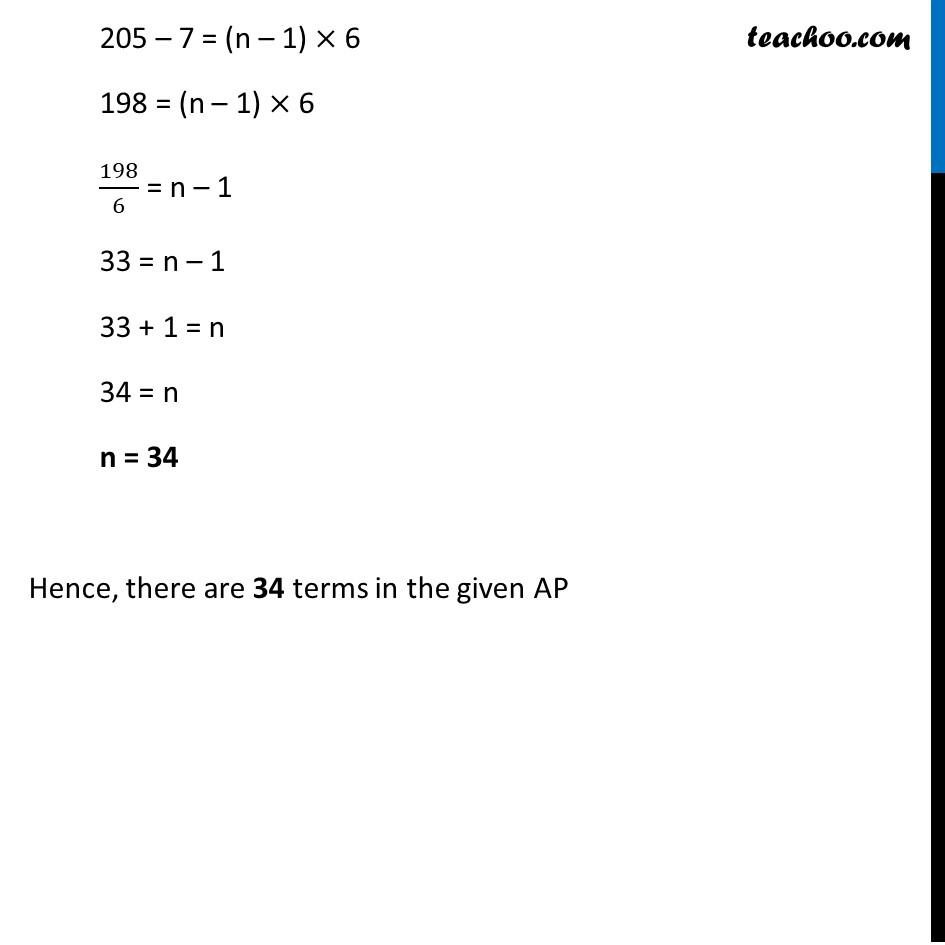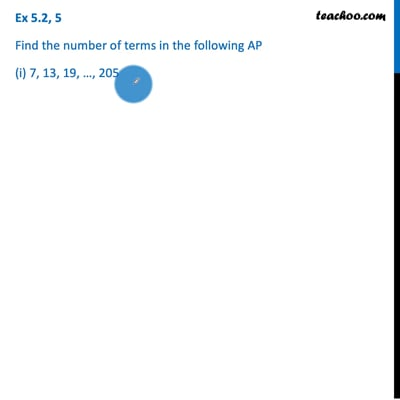Finding n

Chapter 5 Class 10 Arithmetic Progressions (Term 2)
Concept wiseThis video is only available for Teachoo black users

### Transcript

Ex 5.2, 5 Find the number of terms in the following AP (i) 7, 13, 19, …, 205 Given AP 7, 13, 19, … 205 Here, a = 7 d = 13 – 7 = 6 an = 205 We need to find n an = a + (n – 1) d 205 = 7 + (n – 1) × 6 205 – 7 = (n – 1) × 6 198 = (n – 1) × 6 198/6 = n – 1 33 = n – 1 33 + 1 = n 34 = n n = 34 Hence, there are 34 terms in the given AP